### Home > CC2 > Chapter 8 > Lesson 8.3.4 > Problem8-106

8-106.

Sketch each angle below on your paper, and label each one with its measure in degrees.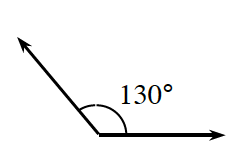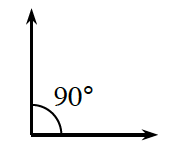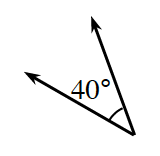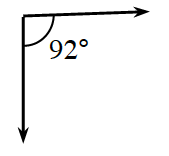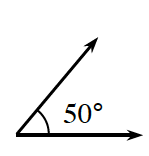1. Identify whether each angle is an acute, obtuse, or right angle.

Remember:
1) Right angles are $90°$.
2) Acute angles are less than $90°$.
3) Obtuse angles are greater than $90°$, and less than $180°$.

2. Which angles could form a complementary pair?

Complementary angles add to $90°$.

$40°+50°=90°$. Thus, $40°$ and $50°$ are a complementary pair.

3. Which angles could form a supplementary pair?

Supplementary angles add to $180°$.